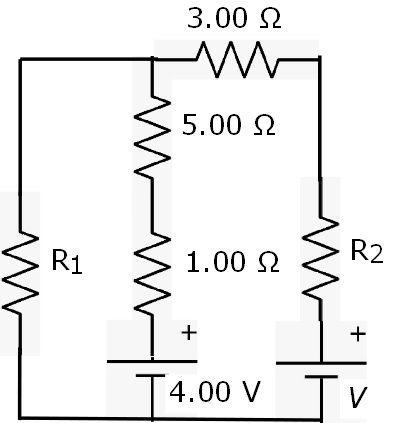# The circuit shown is connected for 1.70 min. Assume R1 = 8.70 ohm, R2 = 2.00 ohm, and V = 11.0 V....

## Question:

The circuit shown is connected for 1.70 min. Assume R1 = 8.70 {eq}\Omega {/eq}, R2 = 2.00 {eq}\Omega {/eq}, and V = 11.0 V.

1) Determine the current and direction in the left, middle and right branches of the circuit.

2) Find the energy delivered by each battery.

3) Find the energy delivered to each resistor.## Kirchoff's law:

Kirchhoffs Circuit Laws allow us to solve complex circuit problems by defining a set of basic network laws and theorems for the voltages and currents around a circuit

Applying Kirchoff's law to the right loop

{eq}V =R_2I +3I+5I_1+I_1+4 {/eq}

{eq}11-4 = 2I+3I+5I_1 + I_1 {/eq}

{eq}7 = 5I+6I_1 {/eq}

. ---1

Appl...

Become a Study.com member to unlock this answer! Create your account